### IMO Shortlist 1999 problem G4

Kvaliteta:
Avg: 0,0
Težina:
Avg: 7,0
For a triangle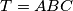$T = ABC$ we take the point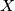$X$ on the side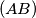$(AB)$ such that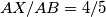$AX/AB=4/5$, the point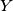$Y$ on the segment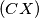$(CX)$ such that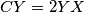$CY = 2YX$ and, if possible, the point$Z$ on the ray (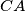$CA$ such that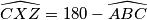$\widehat{CXZ} = 180 - \widehat{ABC}$. We denote by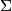$\Sigma$ the set of all triangles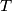$T$ for which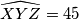$\widehat{XYZ} = 45$. Prove that all triangles from$\Sigma$ are similar and find the measure of their smallest angle.
Izvor: Međunarodna matematička olimpijada, shortlist 1999